### IMO Shortlist 2018 problem A3

Kvaliteta:
Avg: 0,0
Težina:
Avg: 7,0

Given any set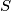$S$ of postive integers, show that at least one of the following two assertions holds:

(1) There exist distinct finite subsets$F$ and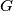$G$ of$S$ such that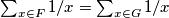$\sum_{x\in F}1/x=\sum_{x\in G}1/x$;

(2) There exists a positive rational number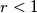$r<1$ such that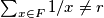$\sum_{x\in F}1/x\neq r$ for all finite subsets$F$ of$S$.

Izvor: https://www.imo-official.org/problems/IMO2018SL.pdf